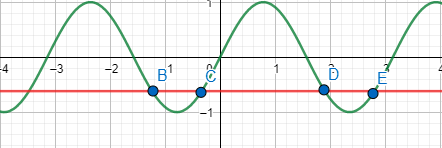Courses
Courses for Kids
Free study material
Free LIVE classes
More# To find the number of solutions of the equation $\sin \theta +\cos \theta =\sin 2\theta$ in the interval $[\pi ,-\pi ]$-(a) 1(b) 2(c) 3(d) 4

Last updated date: 23rd Mar 2023
Total views: 305.7k
Views today: 5.85kVerified
305.7k+ views
Hint: Since, we have different terms (in terms that on LHS, there are trigonometric terms containing $\theta$ and on RHS, there is a $2\theta$ term) on LHS and RHS of the trigonometric equation, we solve this trigonometric equation by squaring LHS and RHS terms. This way we will be able to simplify the equation easily.

Thus, we have,
$\sin \theta +\cos \theta =\sin 2\theta$
Now, squaring the LHS and RHS, we have,
${{(\sin \theta +\cos \theta )}^{2}}={{(\sin 2\theta )}^{2}}$
${{(\sin \theta )}^{2}}+{{(\cos \theta )}^{2}}+2\sin \theta \cos \theta ={{(\sin 2\theta )}^{2}}$
1+$\sin 2\theta ={{(\sin 2\theta )}^{2}}$ -- (1)
Since, ${{(\sin \theta )}^{2}}+{{(\cos \theta )}^{2}}=1$ and $2\sin \theta \cos \theta =\sin 2\theta$
Now, solving (1) further,
1+$\sin 2\theta ={{(\sin 2\theta )}^{2}}$
Let $\sin 2\theta$=t, thus, we have,
1+$t={{t}^{2}}$
${{t}^{2}}-t-1=0$-- (2)
To solve, $a{{x}^{2}}+bx+c=0$, the solution is-
x=$\dfrac{-b\pm \sqrt{{{b}^{2}}-4ac}}{2a}$
Now solving the equation (2), we have,
t= $\dfrac{1\pm \sqrt{{{1}^{2}}-4(-1)(1)}}{2(1)}$
t=$\dfrac{1\pm \sqrt{5}}{2}$
Now, we can eliminate, t=$\dfrac{1+\sqrt{5}}{2}$=1.618
Since, t=$\sin 2\theta$ would be greater than 1. This would not be possible since, -1$\le $$\sin 2\theta$$\le$1.
Thus, we only have one solution,
t=$\dfrac{1-\sqrt{5}}{2}$
Since, t=$\sin 2\theta$, we have,
$\sin 2\theta$=$\dfrac{1-\sqrt{5}}{2}$
Now, to solve this equation, we will make use of graph,Now, to get the number of solutions, we have to find the number of intersection of y=$\dfrac{1-\sqrt{5}}{2}$and y=$\sin 2\theta$ between [$\pi ,-\pi$]. (Also, for reference, $\pi$is approximately 3.14)
Clearly, we see that there are four intersections within the range [$\pi ,-\pi$].
Hence, there are four solutions to the trigonometric equation $\sin \theta +\cos \theta =\sin 2\theta$.

Note: While solving trigonometric equations, we should try to solve the question by bringing LHS and RHS in same degree of angle (that is, in this case, by squaring the LHS and RHS terms, we were eventually able to bring both LHS and RHS in terms of $2\theta$. Finally, we should ensure that the solution is always within the limits [-1,1] since, -1$\le $$\sin x$$\le$1.